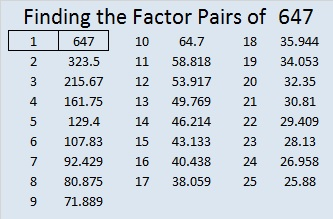# 647 and Level 4

647 is the sum of the five prime numbers from 113 to 139.

647 appears in only one Pythagorean triple, the primitive 647-209304-209305.Print the puzzles or type the solution on this excel file: 10 Factors 2015-10-12

—————————————————————————————————

• 647 is a prime number.
• Prime factorization: 647 is prime.
• The exponent of prime number 647 is 1. Adding 1 to that exponent we get (1 + 1) = 2. Therefore 647 has exactly 2 factors.
• Factors of 647: 1, 647
• Factor pairs: 647 = 1 x 647
• 647 has no square factors that allow its square root to be simplified. √647 ≈ 25.43619.How do we know that 647 is a prime number? If 647 were not a prime number, then it would be divisible by at least one prime number less than or equal to √647 ≈ 25.4. Since 647 cannot be divided evenly by 2, 3, 5, 7, 11, 13, 17, 19, or 23, we know that 647 is a prime number.

—————————————————————————————————This site uses Akismet to reduce spam. Learn how your comment data is processed.